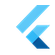Flutter Engineflutter::MatrixDecomposition Class Reference

`#include <matrix_decomposition.h>`

## Public Member Functions

MatrixDecomposition (const SkMatrix &matrix)

MatrixDecomposition (SkM44 matrix)

~MatrixDecomposition ()

bool IsValid () const

const SkV3 & translation () const

const SkV3 & scale () const

const SkV3 & shear () const

const SkV4 & perspective () const

const SkV4 & rotation () const

## Detailed Description

Decomposes a given non-degenerate transformation matrix into a sequence of operations that produced it. The validity of the decomposition must always be checked before attempting to access any of the decomposed elements.

Definition at line 17 of file matrix_decomposition.h.

## ◆ MatrixDecomposition() [1/2]

 flutter::MatrixDecomposition::MatrixDecomposition ( const SkMatrix & matrix )

Definition at line 16 of file matrix_decomposition.cc.

17  : MatrixDecomposition(SkM44{matrix}) {}
MatrixDecomposition(const SkMatrix &matrix)

## ◆ MatrixDecomposition() [2/2]

 flutter::MatrixDecomposition::MatrixDecomposition ( SkM44 matrix )

Definition at line 28 of file matrix_decomposition.cc.

References flutter::SkV3Combine(), flutter::SkV3Normalize(), and ~MatrixDecomposition().

28  : valid_(false) {
29  if (matrix.rc(3, 3) == 0) {
30  return;
31  }
32
33  for (int i = 0; i < 4; i++) {
34  for (int j = 0; j < 4; j++) {
35  matrix.setRC(j, i, matrix.rc(j, i) / matrix.rc(3, 3));
36  }
37  }
38
39  SkM44 perpective_matrix = matrix;
40  for (int i = 0; i < 3; i++) {
41  perpective_matrix.setRC(3, i, 0.0);
42  }
43
44  perpective_matrix.setRC(3, 3, 1.0);
45
46  SkM44 inverted(SkM44::Uninitialized_Constructor::kUninitialized_Constructor);
47  if (!perpective_matrix.invert(&inverted)) {
48  return;
49  }
50
51  if (matrix.rc(3, 0) != 0.0 || matrix.rc(3, 1) != 0.0 ||
52  matrix.rc(3, 2) != 0.0) {
53  const SkV4 right_hand_side = matrix.row(3);
54
55  perspective_ = inverted.transpose() * right_hand_side;
56
57  matrix.setRow(3, {0, 0, 0, 1});
58  }
59
60  translation_ = {matrix.rc(0, 3), matrix.rc(1, 3), matrix.rc(2, 3)};
61
62  matrix.setRC(0, 3, 0.0);
63  matrix.setRC(1, 3, 0.0);
64  matrix.setRC(2, 3, 0.0);
65
66  SkV3 row;
67  for (int i = 0; i < 3; i++) {
68  row[i] = {matrix.rc(0, i), matrix.rc(1, i), matrix.rc(2, i)};
69  }
70
71  scale_.x = row.length();
72
73  SkV3Normalize(&row);
74
75  shear_.x = row.dot(row);
76  row = SkV3Combine(row, 1.0, row, -shear_.x);
77
78  scale_.y = row.length();
79
80  SkV3Normalize(&row);
81
82  shear_.x /= scale_.y;
83
84  shear_.y = row.dot(row);
85  row = SkV3Combine(row, 1.0, row, -shear_.y);
86  shear_.z = row.dot(row);
87  row = SkV3Combine(row, 1.0, row, -shear_.z);
88
89  scale_.z = row.length();
90
91  SkV3Normalize(&row);
92
93  shear_.y /= scale_.z;
94  shear_.z /= scale_.z;
95
96  if (row.dot(row.cross(row)) < 0) {
97  scale_ *= -1;
98
99  for (int i = 0; i < 3; i++) {
100  row[i] *= -1;
101  }
102  }
103
104  rotation_.x = 0.5 * sqrt(fmax(1.0 + row.x - row.y - row.z, 0.0));
105  rotation_.y = 0.5 * sqrt(fmax(1.0 - row.x + row.y - row.z, 0.0));
106  rotation_.z = 0.5 * sqrt(fmax(1.0 - row.x - row.y + row.z, 0.0));
107  rotation_.w = 0.5 * sqrt(fmax(1.0 + row.x + row.y + row.z, 0.0));
108
109  if (row.y > row.z) {
110  rotation_.x = -rotation_.x;
111  }
112  if (row.z > row.x) {
113  rotation_.y = -rotation_.y;
114  }
115  if (row.x > row.y) {
116  rotation_.z = -rotation_.z;
117  }
118
119  valid_ = true;
120 }
static SkV3 SkV3Combine(const SkV3 &a, float a_scale, const SkV3 &b, float b_scale)
static void SkV3Normalize(SkV3 *v)

## ◆ ~MatrixDecomposition()

 flutter::MatrixDecomposition::~MatrixDecomposition ( )
default

Referenced by MatrixDecomposition().

## ◆ IsValid()

 bool flutter::MatrixDecomposition::IsValid ( ) const

Definition at line 124 of file matrix_decomposition.cc.

Referenced by flutter::RasterCache::Prepare(), and flutter::testing::TEST().

124  {
125  return valid_;
126 }

## ◆ perspective()

 const SkV4& flutter::MatrixDecomposition::perspective ( ) const
inline

Definition at line 33 of file matrix_decomposition.h.

33 { return perspective_; }

## ◆ rotation()

 const SkV4& flutter::MatrixDecomposition::rotation ( ) const
inline

Definition at line 35 of file matrix_decomposition.h.

Referenced by flutter::testing::TEST().

35 { return rotation_; }

## ◆ scale()

 const SkV3& flutter::MatrixDecomposition::scale ( ) const
inline

Definition at line 29 of file matrix_decomposition.h.

Referenced by flutter::SkV3Normalize(), and flutter::testing::TEST().

29 { return scale_; }

## ◆ shear()

 const SkV3& flutter::MatrixDecomposition::shear ( ) const
inline

Definition at line 31 of file matrix_decomposition.h.

31 { return shear_; }

## ◆ translation()

 const SkV3& flutter::MatrixDecomposition::translation ( ) const
inline

Definition at line 27 of file matrix_decomposition.h.

Referenced by flutter::testing::TEST().

27 { return translation_; }

The documentation for this class was generated from the following files: